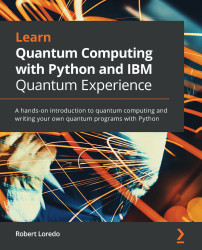•#### Learn Quantum Computing with Python and IBM Quantum Experience#### Overview of this book

IBM Quantum Experience is a platform that enables developers to learn the basics of quantum computing by allowing them to run experiments on a quantum computing simulator and a real quantum computer. This book will explain the basic principles of quantum mechanics, the principles involved in quantum computing, and the implementation of quantum algorithms and experiments on IBM's quantum processors. You will start working with simple programs that illustrate quantum computing principles and slowly work your way up to more complex programs and algorithms that leverage quantum computing. As you build on your knowledge, you’ll understand the functionality of IBM Quantum Experience and the various resources it offers. Furthermore, you’ll not only learn the differences between the various quantum computers but also the various simulators available. Later, you’ll explore the basics of quantum computing, quantum volume, and a few basic algorithms, all while optimally using the resources available on IBM Quantum Experience. By the end of this book, you'll learn how to build quantum programs on your own and have gained practical quantum computing skills that you can apply to your business.
PrefaceSection 1: Tour of the IBM Quantum Experience (QX)Free Chapter
Chapter 1: Exploring the IBM Quantum ExperienceChapter 2: Circuit Composer – Creating a Quantum CircuitChapter 3: Creating Quantum Circuits using Quantum Lab NotebooksSection 2: Basics of Quantum ComputingChapter 4: Understanding Basic Quantum Computing PrinciplesChapter 5: Understanding the Quantum Bit (Qubit)Chapter 6: Understanding Quantum Logic GatesSection 3: Algorithms, Noise, and Other Strange Things in Quantum WorldChapter 7: Introducing Qiskit and its ElementsChapter 8: Programming with Qiskit TerraChapter 9: Monitoring and Optimizing Quantum CircuitsChapter 10: Executing Circuits Using Qiskit AerChapter 11: Mitigating Quantum Errors Using IgnisChapter 12: Learning about Qiskit AquaChapter 13: Understanding Quantum AlgorithmsChapter 14: Applying Quantum AlgorithmsAssessmentsOther Books You May EnjoyAppendix A: Resources# Summary

In this chapter, we covered some of the many quantum algorithms that employ common techniques that are used in a variety of other quantum algorithms.

The goal of this chapter was to explore each of them systematically so you can have a good combination of understanding of the problem each algorithm is solving. Also, now you have an understanding of how to implement them on both a simulator and a quantum computer. The topics here are, of course, foundational and oracle-based, although the techniques are commonly found in many other quantum algorithms.

In the next chapter, we will step away from the oracle-based foundational algorithms and look at another form of algorithm that solves similar problems. However, rather than using phases to identify the solution, it will instead leverage periodicity, which is primarily why they are called periodic algorithms.